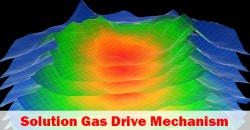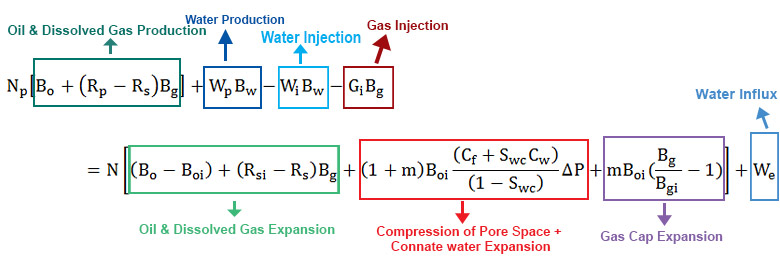## Solution Gas Drive Mechanism Explained in Material Balance EquationMaterial balance equation can be applied for any drive mechanism and this article demonstrates how to apply the material balance equation in a solution drive mechanism. For a solution drive mechanism, there are 2 cases. The first case is when reservoir pressure is above a bubble point and the second case is when reservoir pressure is below a bubble point.

Solution Gas above Bubble Point